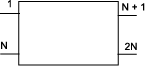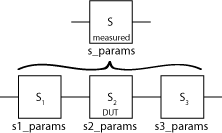# deembedsparams

De-embed 2N-port S-parameters

## Syntax

`` s2_params = deembedsparams(s_params,s1_params,s3_params)``
``hs2 = deembedsparams(hs,hs1,hs3)``

## Description

example

```` s2_params = deembedsparams(s_params,s1_params,s3_params)` de-embeds `s2_params` from cascaded S-parameters `s_params`, by removing the effects of `s1_params` and `s3_params`. `deembedsparams` assumes that you are using the port ordering shown here:This function is ideal for situations in which the S-parameters of a `DUT` (device under test) must be de-embedded from S-parameters obtained through measurement.```
````hs2 = deembedsparams(hs,hs1,hs3)` de-embeds S-parameter object, `hs2` from the chain `hs`. ```

## Examples

collapse all

Read measured S-parameters of the cascaded network from `cascadedbackplanes.s4p`

```S_measuredBJT = sparameters('cascadedbackplanes.s4p'); freq = S_measuredBJT.Frequencies;```

Calculate the S-parameters of the left fixture of the network.

```leftpad = circuit('left'); add(leftpad,[1 2],inductor(1e-9)) add(leftpad,[2 3],capacitor(100e-15)) setports(leftpad,[1 0],[3 0],[2 0],[3 0]) S_leftpad = sparameters(leftpad,freq)```
```S_leftpad = sparameters: S-parameters object NumPorts: 4 Frequencies: [1496x1 double] Parameters: [4x4x1496 double] Impedance: 50 rfparam(obj,i,j) returns S-parameter Sij ```

Calculate the S-parameters of the right fixture of the network.

```rightpad = circuit('right'); add(rightpad,[1 3],capacitor(100e-15)) add(rightpad,[1 2],inductor(1e-9)) setports(rightpad,[1 0],[3 0],[2 0],[3 0]) S_rightpad = sparameters(rightpad,freq)```
```S_rightpad = sparameters: S-parameters object NumPorts: 4 Frequencies: [1496x1 double] Parameters: [4x4x1496 double] Impedance: 50 rfparam(obj,i,j) returns S-parameter Sij ```

De-embed the S-parameters of the DUT. The output is stored in `S-DUT` in MATLAB® workspace.

`S_DUT = deembedsparams(S_measuredBJT,S_leftpad,S_rightpad)`
```S_DUT = sparameters: S-parameters object NumPorts: 4 Frequencies: [1496x1 double] Parameters: [4x4x1496 double] Impedance: 50 rfparam(obj,i,j) returns S-parameter Sij ```

## Input Arguments

collapse all

S-parameter data, specified as `2Nx2NxK` arrays of `K` 2N-port S-parameters. `s_params` is the measured S-parameter array of the cascaded network. `s1_params` represents the first network of the cascade, and `s3_params` represents the third network. The function assumes that all networks in the cascade have the same reference impedance and are measured at the same frequencies. The function assumes the configuration of the cascade shown here:Data Types: `double`
Complex Number Support: Yes

S-parameter objects, specified as 2N-port scalar handle objects, which can include numeric arrays of S-parameters. The function checks that the `Frequencies` and `Impedance` properties are the same for all three inputs.

Data Types: `function_handle`

## Output Arguments

collapse all

S-parameter data, returned as `2Nx2NxK` arrays of `K` 2N-port s-parameters, containing de-embedded S-parameters of the DUT (device under test).

Data Types: `double`
Complex Number Support: Yes

S-parameter objects, returned as 2N-port scalar handle objects, containing de-embedded S-parameter objects of `DUT` (device under test).

Data Types: `function_handle`

## Version History

Introduced before R2006a